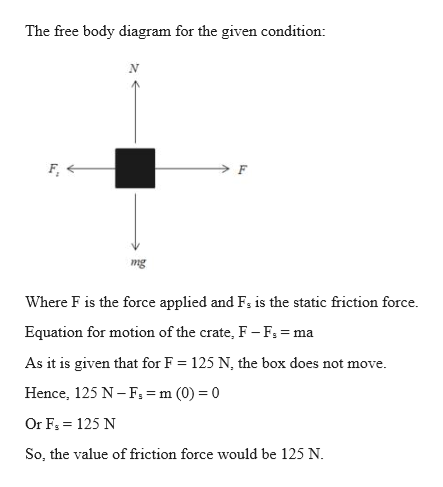# A man exerts a horizontal force of 125 N on a crate with amass of 30.0 kg. (a) If the crate doesn’t move, what’s the magnitudeof the static friction force? (b) What is the minimumpossible value of the coefficient of static friction between thecrate and the floor?

Question
56 views

A man exerts a horizontal force of 125 N on a crate with a
mass of 30.0 kg. (a) If the crate doesn’t move, what’s the magnitude
of the static friction force? (b) What is the minimum
possible value of the coefficient of static friction between the
crate and the floor?

check_circle

Step 1

Given:

Mass of the crate = 30 kg

Force applied by the man = 125 N

Step 2

Calculating the value of...help_outlineImage TranscriptioncloseThe free body diagram for the given condition: F, mg Where F is the force applied and F; is the static friction force. Equation for motion of the crate, F -F; = ma As it is given that for F = 125 N, the box does not move. %3D Hence, 125 N-F: = m (0) = 0 Or F, = 125 N So, the value of friction force would be 125 N. fullscreen

### Want to see the full answer?

See Solution

#### Want to see this answer and more?

Solutions are written by subject experts who are available 24/7. Questions are typically answered within 1 hour.*

See Solution
*Response times may vary by subject and question.
Tagged in

### Physics